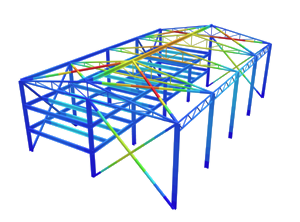SkyCiv Documentation

Your guide to SkyCiv software - tutorials, how-to guides and technical articles

1. Home
2. Tutorials
3. Section Tutorials
4. Calculating the Statical or First Moment of Area of Beam Sections

# Calculating the Statical or First Moment of Area of Beam Sections

## How to Calculate the Statical or First Moment of Area of Beam Sections

The statical or first moment of area (Q) simply measures the distribution of a beam sections’s area relative to an axis. It is calculated by taking the summation of all areas, multiplied by its distance from a particular axis (Area by Distance).

In fact, you may not have realised it, but if you’ve calculated the centroid of a beam section then you would have already calculated the first moment of area. Also this property, often denoted by Q, is most commonly used when determining the shear stress of a beam section.

Since beam sections are usually made up many geometries we first need to split the section into segments. After this, the area and centroid of each segment is calculated to find the overall statical moment of area.

Consider the I-beam section shown below. In our previous tutorial we already found the centroid to be 216.29 mm from the bottom of the section. To calculate the statical moment of area relative to the horizontal x-axis, the section can be split into 4 segments as illustrated: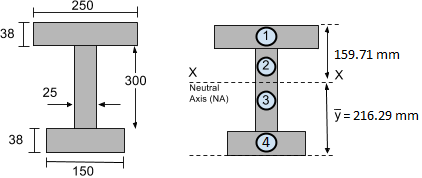Remember that the first moment of area is the summation of the areas multiplied by the distance from the axis. So the formula for the statical moment of area relative to the horizontal x-axis is:Now for a result such as shear stress we often want the statical moment of either the TOP or BOTTOM of the section relative to the Neutral Axis (NA) XX. Let’s start with the TOP portion of the section (i.e. Segments 1 and 2). We’ll find Ai and yi for each segment of the I-beam section above the neutral axis and then compute the statical moment of area (Qx). Remember we measure the distances from the neutral axis!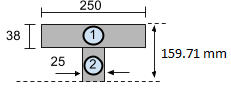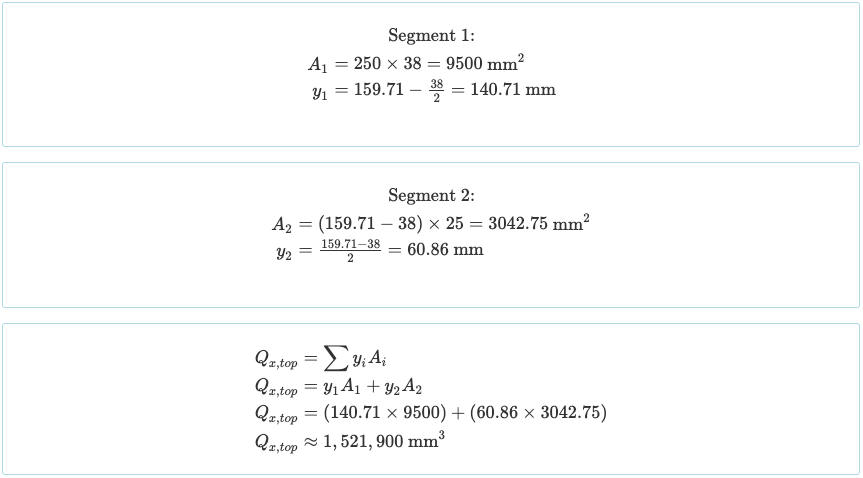Similarly, we can calculate the statical moment of area of the BOTTOM portion of the section. This involves Segments 3 and 4 which are below the neutral axis.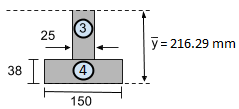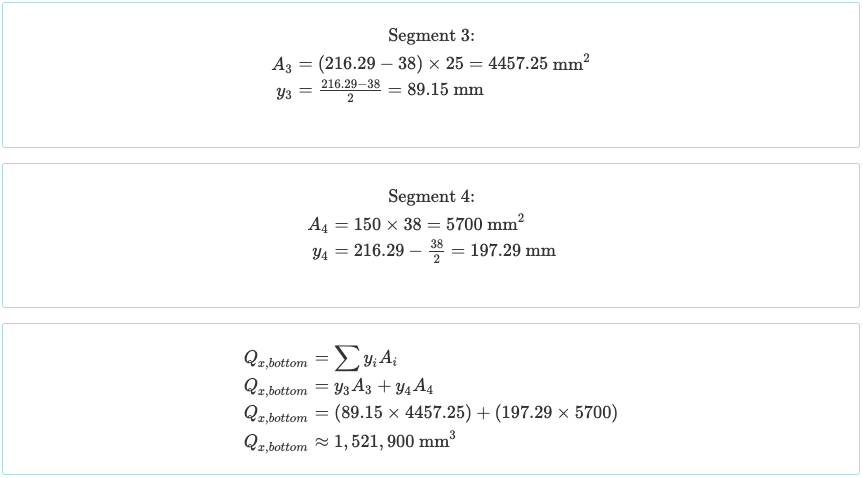What you’ll notice is that the statical moment of area above the neutral axis is equal to that below the neutral axis!

[math]
{Q}_{x,top}={Q}_{x,bottom}
[math]

Of course you don’t need to do all these calculations manually because you can use our fantastic Free Moment of Inertia Calculator to find the statical moment of area of beam sections.

Visit the next step: How to Calculate the Moment of Inertia of a Beam Section.